Ecological Archives E094-131-A1

Joseph M. Northrup, Mevin B. Hooten, Charles R. Anderson Jr., George Wittemyer. 2013. Practical guidance on characterizing availability in resource selection functions under a use–availability design. Ecology 94:1456–1463. http://dx.doi.org/10.1890/12-1688.1

Appendix A. Simulation of landscape covariates as Gaussian random fields.

To assess how resource selection function (RSF) coefficient estimates were  influenced by the interaction between spatial autocorrelation in environmental covariates and the size and spatial extent of the availability sample we fit RSFs to data simulated from environmental covariates that were themselves simulated as a Gaussian random field, using the grf function in the package 'geoR':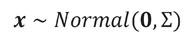(A.1)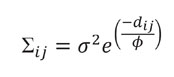(A.2)

where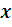is a simulated environmental covariate, Σ is a covariance matrix,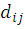is the distance between cellsand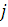, and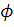is the range parameter controlling the level of correlation among cells. At larger values ofthe landscape is more spatially autocorrelated, while small values produce a more random landscape (Fig. A1). We set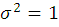and variedfrom 0.001 to 100 (0.001, 0.05, 1, 2.5, 5, 10, 20, 40, 100). Using these covariates we simulated used data as an inhomogeneous Poisson spatial point process, and fit RSFs with both matched and mismatched availability samples (see main text). Results are presented in Figs. A2–A4.

The above analysis provided an assessment of how autocorrelation interacts with the size and spatial extent of the availability sample to influence RSF coefficient estimates for a continuous covariate. For binary covariates, the proportion of the landscape composed of that covariate also has the potential to influence this interaction. To examine this potential we again simulated environmental covariates as a Gaussian random field withparameters of 0.001 and 10. We then converted these covariates to binary covariates by selecting a threshold above which all values were converted to 1s and below which they were converted to 0s. We chose thresholds to simulate 2.5%, 25%, and 50% of the landscape being composed of the binary variable (Fig. A6). Using these covariates we simulated used data as an inhomogeneous Poisson spatial point process and fit RSFs with both matched and mismatched availability sample (see main text). Results are presented in Fig. A6.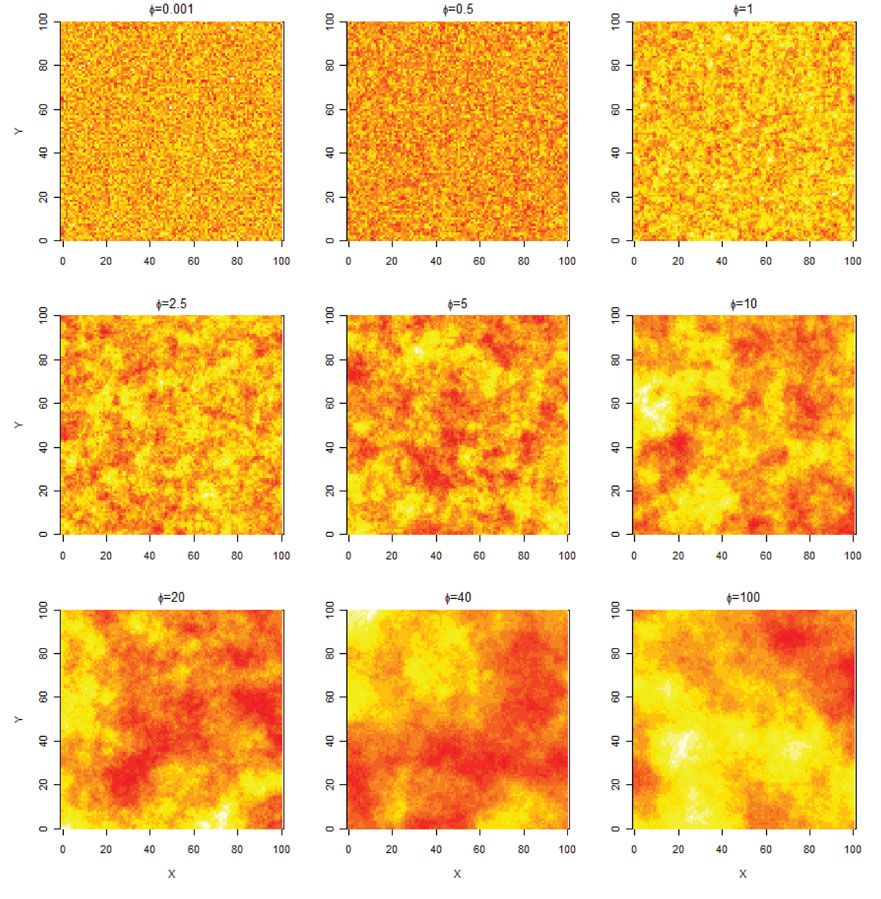Fig. A1. Continuous environmental covariate simulated as a Gaussian random field, with varyingparameters.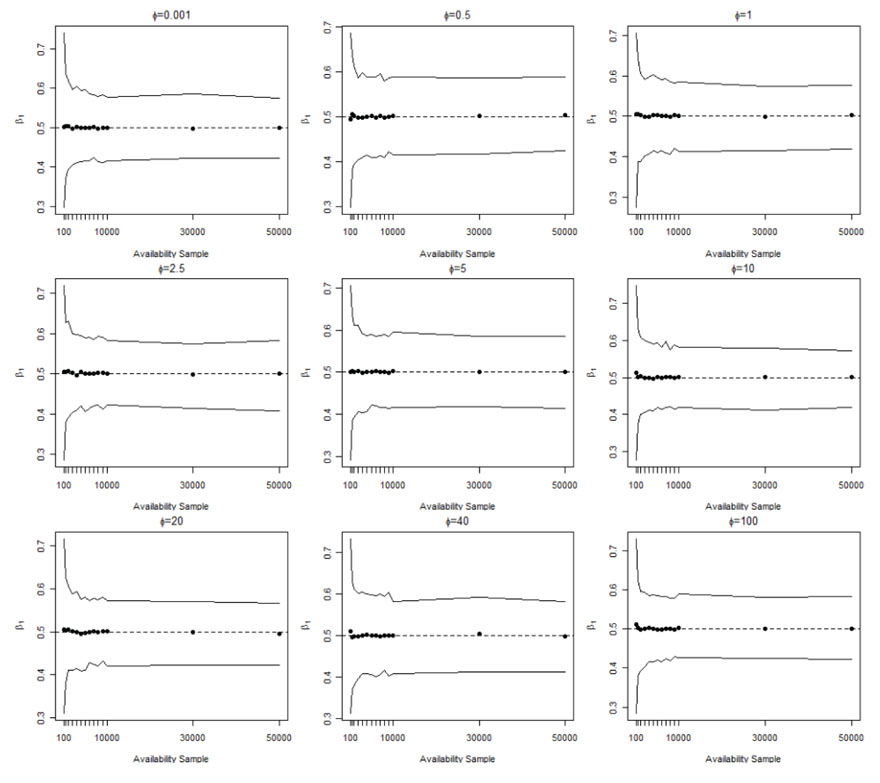Fig. A2. Coefficient estimator (black points) and 95% simulation enevelopes (solid lines) from 500 RSF model iterations fit to data simulated from covariates generated as Gaussian random fields with varyingparameters. Availability was drawn from the same spatial extent as use. Dashed lines represent the coefficient value from which the used data were simulated.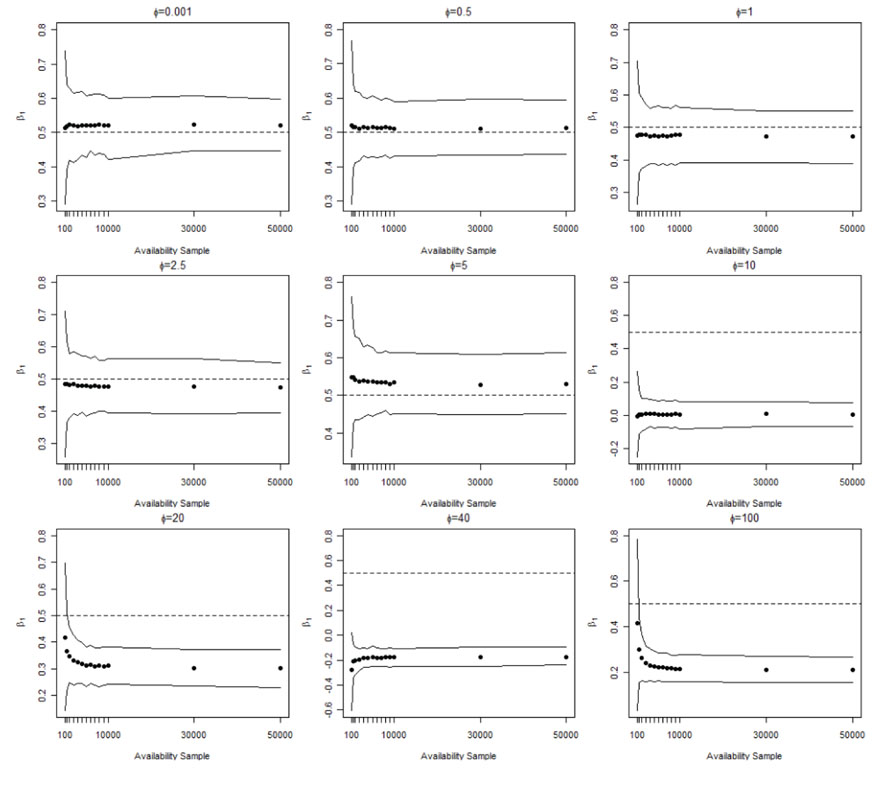Fig. A3. Coefficient estimator (black points) and 95% simulation envelopes (solid lines) from 500 RSF model iterations fit to data simulated from covariates generated as Gaussian random fields with varyingparameters. Availability was drawn from a different spatial extent as use. Dashed lines represent the coefficient value from which the used data were simulated. Approximately 600 used locations were simulated for each iteration.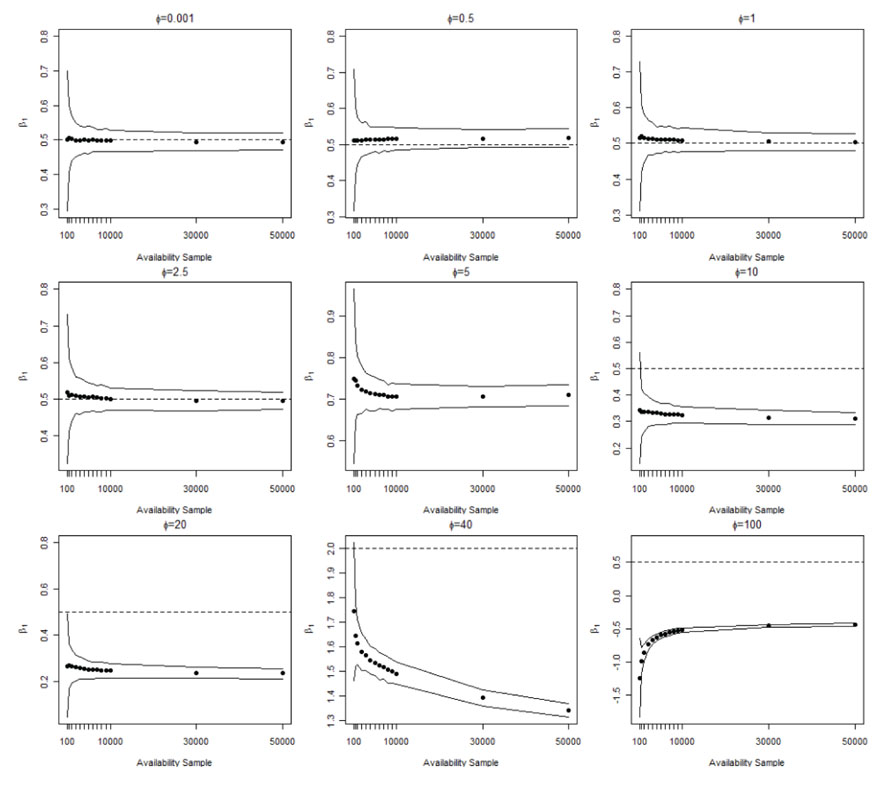Fig. A4. Coefficient estimator (black points) and 95% simulation envelopes (solid lines) from 500 RSF model iterations fit to data simulated from covariates generated as Gaussian random fields with varyingparameters. Availability was drawn from a different spatial extent as use. Dashed lines represent the coefficient value from which the used data were simulated. Approximately 6000 used locations were simulated for each iteration.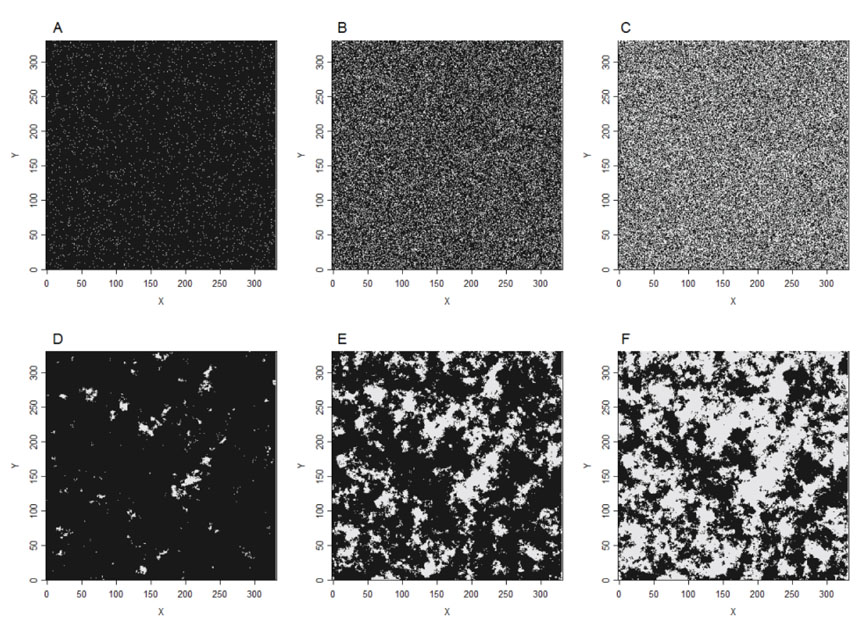Fig. A5. Binary environmental covariate simulated as a Gaussian random field with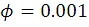(A–C) or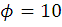(D–F), and converted to a binary covariate composing 2.5% (A and D), 25 % (B and E) or 50% (C and F) of the landscape.